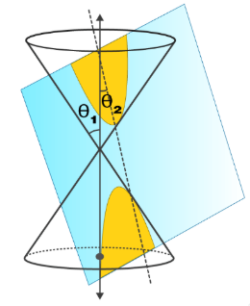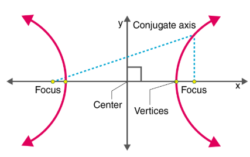# Important Properties of Hyperbola

Hyperbola is a locus of the point in the plane with a constant ratio of the difference between the distance from the two fixed points termed foci. Hyperbola is also known as a mirror image of the parabola. The important properties of hyperbola are well explained in this article.

When a plane is intersected by the right circular cone such that the angle between the plane and the vertical axis is less than the vertical angle, a hyperbola is formed. In hyperbola, the plane cuts the two nappes of the cone, which leads to the formation of two disjoint open curves.## Standard Equation of Hyperbola

The standard equation of the hyperbola is given by:

$\frac{x^2}{a^2}-\frac{y^2}{b^2}=1$

where “a” is the distance between the centre and the vertex. Read More

## Terms Related to Hyperbola

 Focus: They are the two points that define the hyperbola and called foci together. Centre: The intersection point of the transverse and conjugate axis. Also the midpoint of the line which joins the two foci. Transverse axis: A line passing through the foci and also called the major axis of hyperbola. Conjugate axis: A line perpendicular to the transverse axis passing through the centre of the hyperbola. Vertex: The intersection with the transverse axis at two points can be termed as the vertices of the hyperbola.## Properties of Hyperbola

1] The difference between the focal distances on the hyperbola is constant which is the same as the transverse axis given by ||PS – PS’|| = 2a.

2] The relation e1-2 + e2-2 = 1 holds good if e1 and e2 represent the eccentricities of hyperbola.

3] A hyperbola is said to be rectangular or equilateral if the transverse and conjugate axes lengths are the same.

4] A rectangular hyperbola has an eccentricity of √2 which is the same as the length of its latus rectum of the axes.

5] The value of x12/a2 – y12/ b2 = 1 is positive, zero or negative if the point (x1, y1) lies within, on or outside of the hyperbola.

6] There exist two lines which intersect the centre of the hyperbola. The tangents to the centre are called the asymptotes of the hyperbola.

7] The latus rectum of the hyperbola can be defined as the line perpendicular to the transverse axis and passing through any of the foci which are parallel to the conjugate axis. It is given by 2b2/a.

Related Articles:

## Solved Examples for IIT JEE

Example 1: If the latus rectum of a hyperbola is 8 and eccentricity be 3 / √5, then what is the equation of the hyperbola?

Solution:

2b2 / a = 8 and 3/√5 = √(1 + b2) / a2

4 / 5 = b2 / a2

a = 5, b = 2√5.

Hence, the required equation of the hyperbola is (x2 / 25) − (y2 / 20) = 1

4x2 − 5y2 = 100

Example 2: What are the foci of the hyperbola 2x2 − 3y2 = 5?

Solution:

The given equation is 2x2 − 3y2 = 5

x2 / [5/2] − y2 / [5/3] = 1

b2 = a2 (e2 − 1)

⇒ 5 / 3 = 5 / 2(e2 − 1)

e = √[5/ 3]

The foci of hyperbola (± ae , 0)

(± √[5 / 2] * √[5 / 3] , 0) = (± 5/√6, 0)

Example 3: Find the length of the transverse axis of the parabola 3x2 − 4y2 = 32.

Solution:

The given equation may be written as

$\frac{x^2}{32/2}-\frac{y^2}{8}=1$ $\frac{x^2}{[4\sqrt{2}/\sqrt{3}]^2}-\frac{y^2}{[2\sqrt{2}]^2}$

Comparing the given equation with $\frac{x^2}{a^2}-\frac{y^2}{b^2}=1$ , we get

$a^2=[\frac{4\sqrt{2}}{3}]^2$ $a=\frac{4\sqrt{2}}{\sqrt{3}}$

Therefore length of transverse axis of a hyperbola = 2a = $2\times \frac{4\sqrt{2}}{\sqrt{3}}=\frac{8\sqrt{2}}{\sqrt{3}}$

Example 4: Find the distance between the directrices of the hyperbola x = 8secθ, y = 8tanθ.

Solution:

Equation of hyperbola is x = 8secθ , y = 8tanθ

x / 8 = secθ , y / 8 = tanθ

∵sec2θ − tan2θ = 1

x2 / 82 − y2 / 82 = 1

Here a = 8 , b = 8

$e=\sqrt{1+\frac{b^2}{a^2}}=\sqrt{1+\frac{8^2}{8^2}}=\sqrt{1+1}=\sqrt{2}$

Distance between directrices = 2*a / e = [2 × 8] / √2 = 8 √2

Example 5: What is the point of contact of the tangent y = x + 2 to the hyperbola 5x2 − 9y2 = 45?

Solution:

Hyperbola is x2 / 9 − y2 / 5 = 1

Hence, the point of contact is

$(\frac{-9}{\sqrt{9-5}}, \frac{-5}{\sqrt{9-5}}) = (\frac{-9}{2}, \frac{-5}{2})$

Hint: Point (−9 /2, −5 /2) satisfies both the equations.

Test your Knowledge on Hyperbola Properties